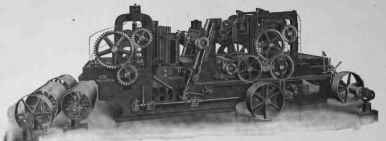1. The allowable pressure on a bearing is 300 pounds per square inch of projected area. What is the required length of the bearing if the total load is 4,500 pounds and the diameter is 3 inches?

2. The cross-head pin of a steam engine must be 2.5 inches in diameter to withstand the shearing strain. If the maximum pressure is 10,000 pounds, what length should be given to the pin?

3. The journals on the tender of a locomotive are 3½X7 inches. The total weight of the tender and load is 60,000 pounds. If there are 8 journals, what is the pressure per square inch of projected area?

4. What horse-power is lost in friction at the circumference of a 3-inch bearing carrying a load of 6,000 pounds, if the number of revolutions per minute is 150 and the coefficient of friction is assumed to be 5 per cent?

5. The cast-iron bracket in Fig. 77 has a load P of 1,000 pounds. Determine the fiber stress in the web section at the base of the bracket if the thickness is taken at ½ inch, and L1 = 12 inches; I = 20 inches; k = 11 inches; t = 1 inch.

6. Calculate the diameter of the bolts at the top of the bracket.

7. Assuming r equal to 6 inches, what is the fiber stress at the root of flange?TIMBER PLANER.

J. A. Fay and Egan Co.